# RD Sharma Solutions for Class 11 Chapter 23 - The Straight Lines Exercise 23.6

This exercise, discusses one of the different forms of the equation of a straight line i.e., the intercept form of a line with illustrations, for better understanding of the topics. Students who find difficulty in solving the exercise wise problems can download the pdf of solutions created by the subject experts at BYJU’S. Students are advised to practice the problems regularly in order to improve their knowledge about the concepts. It will also help them to answer the tricky questions that would appear in the annual exam. For a better understanding of the concepts, RD Sharma Class 11 Maths Solutions pdf is readily available for download, from the links provided below.

## Download the pdf of RD Sharma Solutions for Class 11 Maths Exercise 23.6 Chapter 23 – The Straight Lines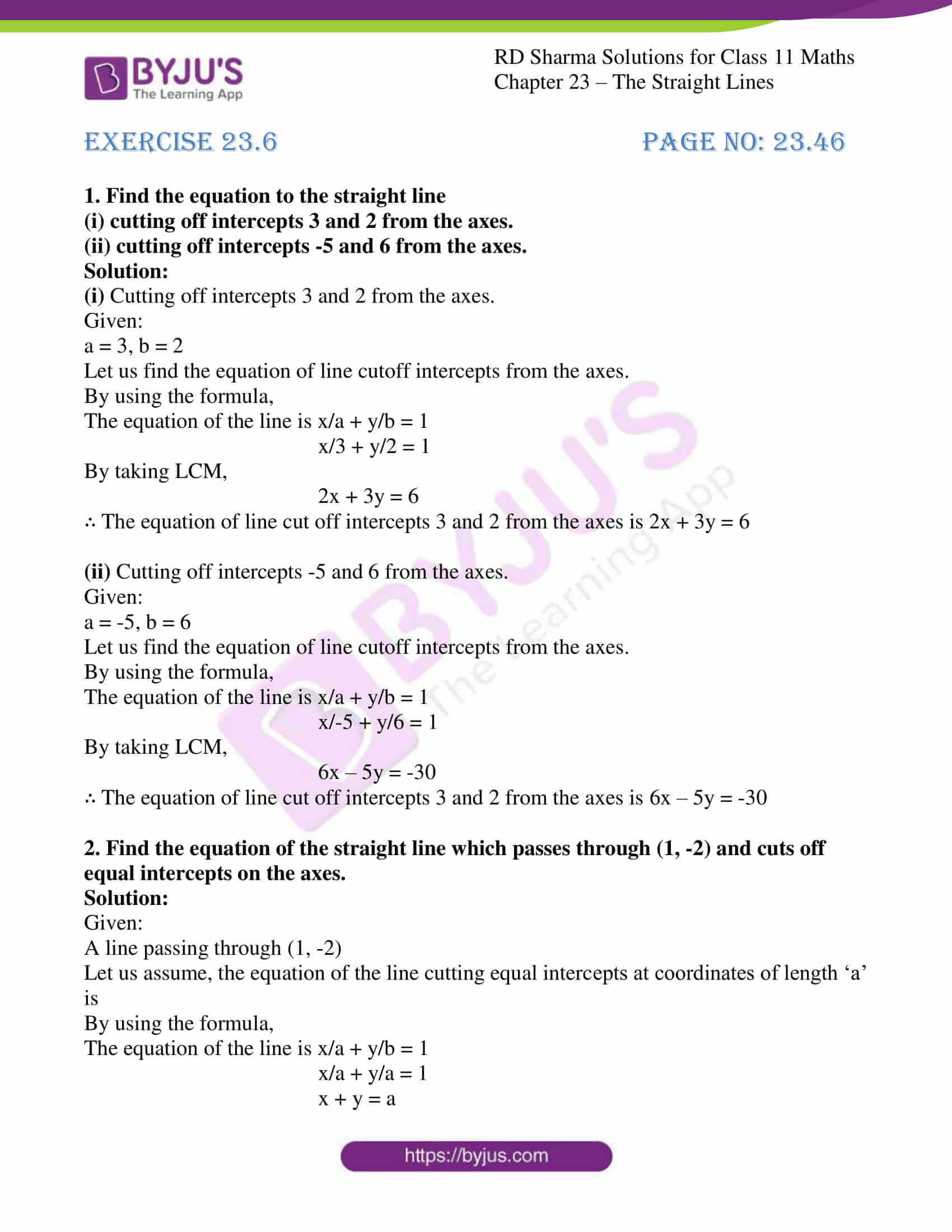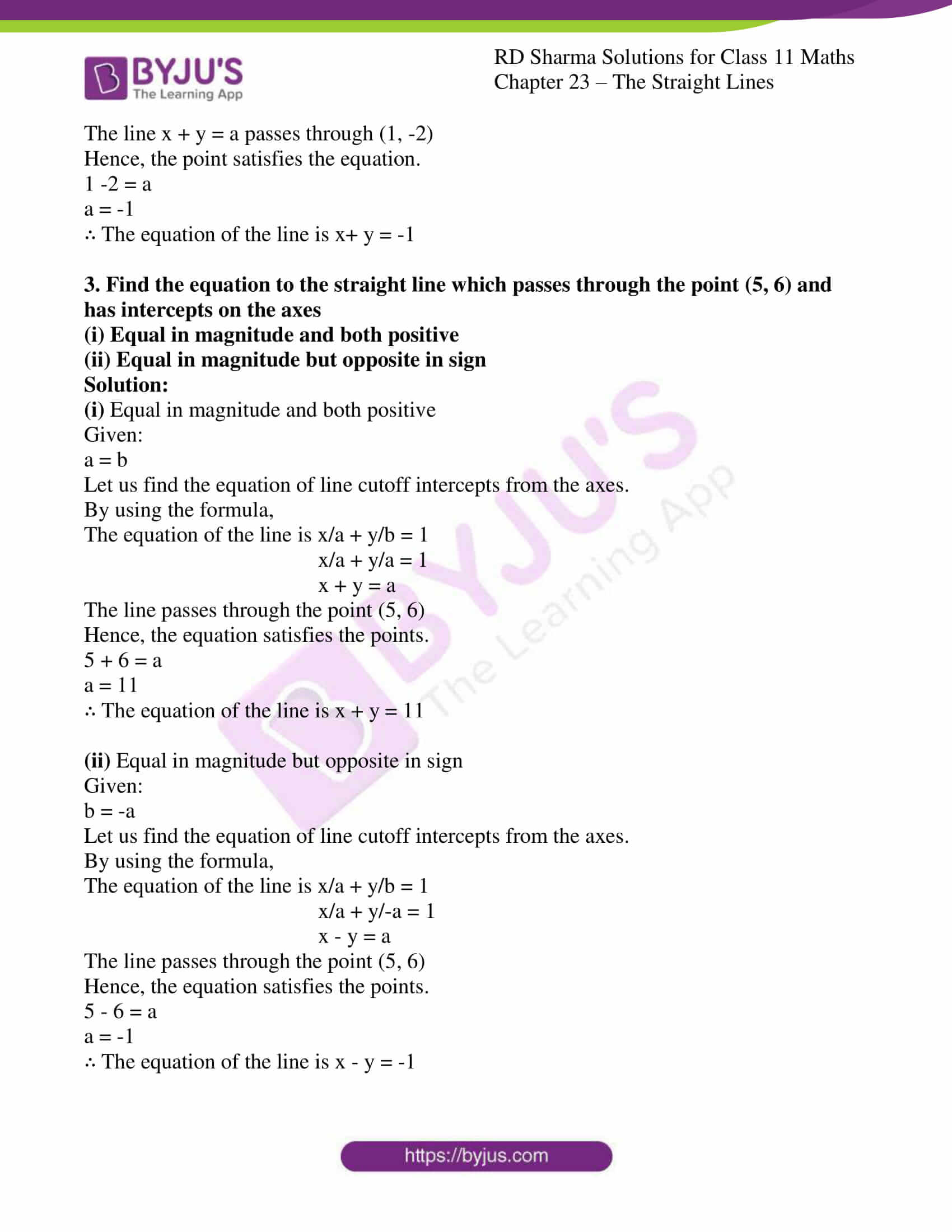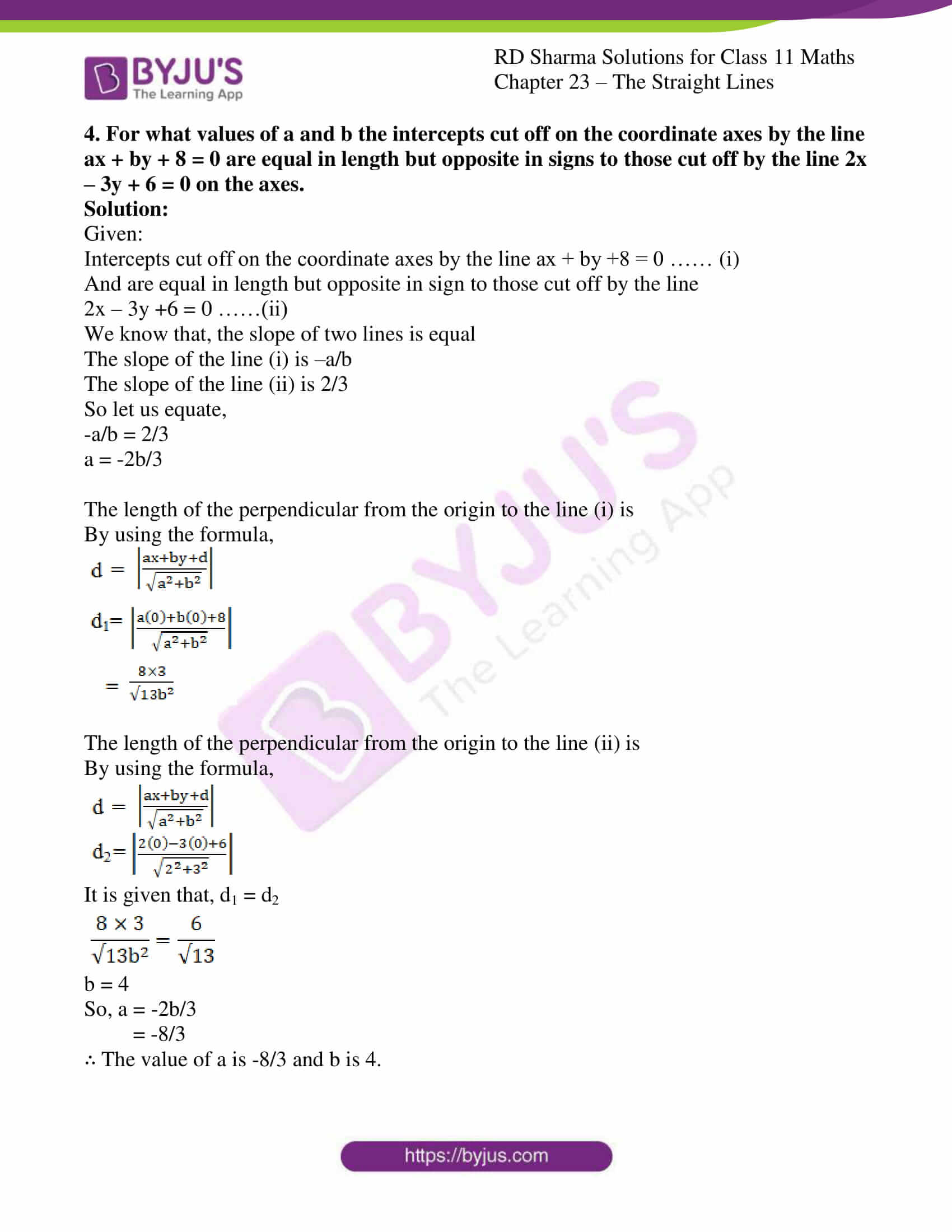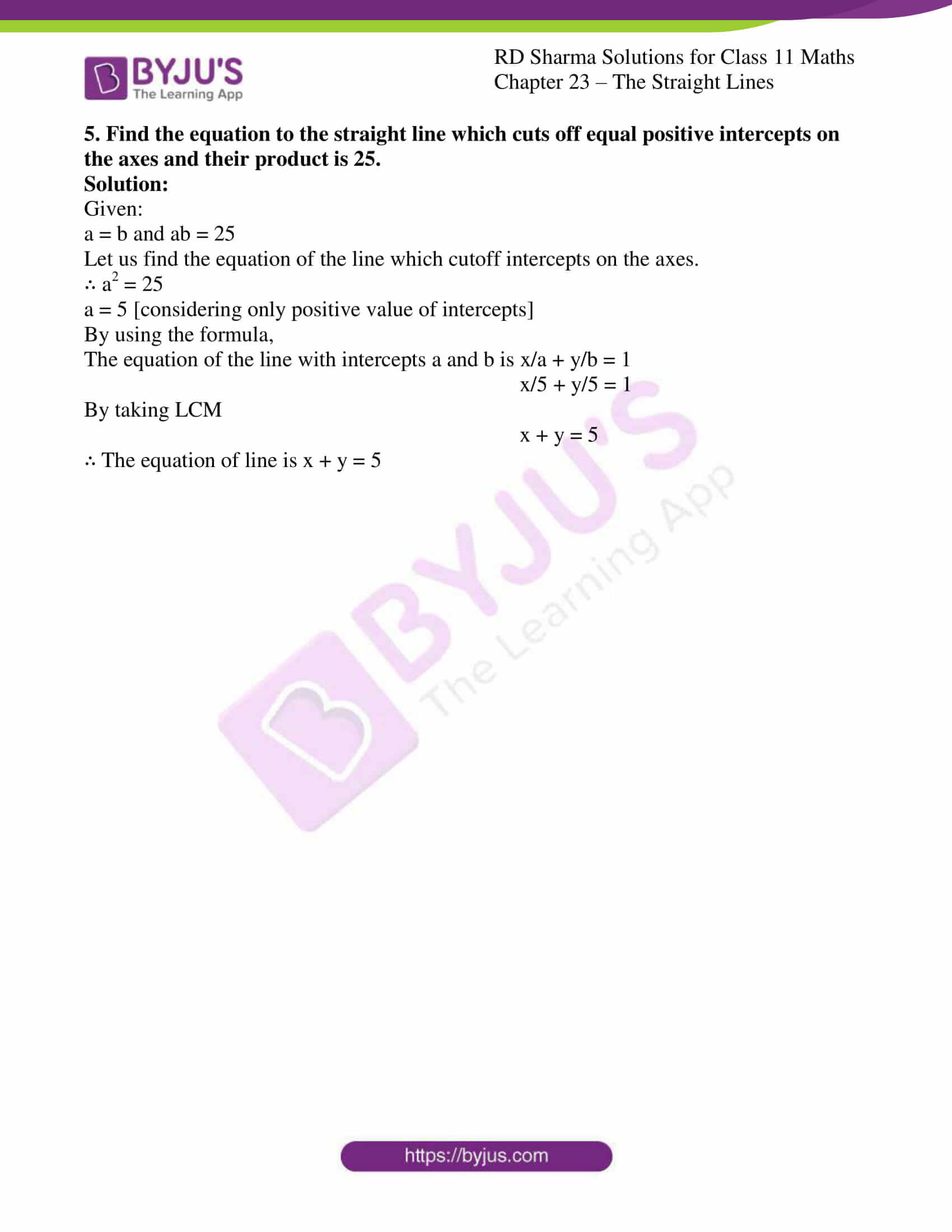### Access answers to RD Sharma Solutions for Class 11 Maths Exercise 23.6 Chapter 23 – The Straight Lines

#### EXERCISE 23.6 PAGE NO: 23.46

1. Find the equation to the straight line
(i) cutting off intercepts 3 and 2 from the axes.

(ii) cutting off intercepts -5 and 6 from the axes.

Solution:

(i) Cutting off intercepts 3 and 2 from the axes.

Given:

a = 3, b = 2

Let us find the equation of line cutoff intercepts from the axes.

By using the formula,

The equation of the line is x/a + y/b = 1

x/3 + y/2 = 1

By taking LCM,

2x + 3y = 6

∴ The equation of line cut off intercepts 3 and 2 from the axes is 2x + 3y = 6

(ii) Cutting off intercepts -5 and 6 from the axes.

Given:

a = -5, b = 6

Let us find the equation of line cutoff intercepts from the axes.

By using the formula,

The equation of the line is x/a + y/b = 1

x/-5 + y/6 = 1

By taking LCM,

6x – 5y = -30

∴ The equation of line cut off intercepts 3 and 2 from the axes is 6x – 5y = -30

2. Find the equation of the straight line which passes through (1, -2) and cuts off equal intercepts on the axes.

Solution:

Given:

A line passing through (1, -2)

Let us assume, the equation of the line cutting equal intercepts at coordinates of length ‘a’ is

By using the formula,

The equation of the line is x/a + y/b = 1

x/a + y/a = 1

x + y = a

The line x + y = a passes through (1, -2)

Hence, the point satisfies the equation.

1 -2 = a

a = -1

∴ The equation of the line is x+ y = -1

3. Find the equation to the straight line which passes through the point (5, 6) and has intercepts on the axes
(i) Equal in magnitude and both positive

(ii) Equal in magnitude but opposite in sign

Solution:

(i) Equal in magnitude and both positive

Given:

a = b

Let us find the equation of line cutoff intercepts from the axes.

By using the formula,

The equation of the line is x/a + y/b = 1

x/a + y/a = 1

x + y = a

The line passes through the point (5, 6)

Hence, the equation satisfies the points.

5 + 6 = a

a = 11

∴ The equation of the line is x + y = 11

(ii) Equal in magnitude but opposite in sign

Given:

b = -a

Let us find the equation of line cutoff intercepts from the axes.

By using the formula,

The equation of the line is x/a + y/b = 1

x/a + y/-a = 1

x – y = a

The line passes through the point (5, 6)

Hence, the equation satisfies the points.

5 – 6 = a

a = -1

∴ The equation of the line is x – y = -1

4. For what values of a and b the intercepts cut off on the coordinate axes by the line ax + by + 8 = 0 are equal in length but opposite in signs to those cut off by the line 2x – 3y + 6 = 0 on the axes.

Solution:

Given:

Intercepts cut off on the coordinate axes by the line ax + by +8 = 0 …… (i)

And are equal in length but opposite in sign to those cut off by the line

2x – 3y +6 = 0 ……(ii)

We know that, the slope of two lines is equal

The slope of the line (i) is –a/b

The slope of the line (ii) is 2/3

So let us equate,

-a/b = 2/3

a = -2b/3

The length of the perpendicular from the origin to the line (i) is

By using the formula,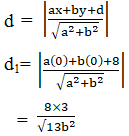The length of the perpendicular from the origin to the line (ii) is

By using the formula,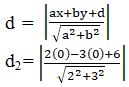It is given that, d1 = d2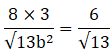b = 4

So, a = -2b/3

= -8/3

∴ The value of a is -8/3 and b is 4.

5. Find the equation to the straight line which cuts off equal positive intercepts on the axes and their product is 25.

Solution:

Given:

a = b and ab = 25

Let us find the equation of the line which cutoff intercepts on the axes.

∴ a2 = 25

a = 5 [considering only positive value of intercepts]

By using the formula,

The equation of the line with intercepts a and b is x/a + y/b = 1

x/5 + y/5 = 1

By taking LCM

x + y = 5

∴ The equation of line is x + y = 5